# 【翻译】Sklearn 与 TensorFlow 机器学习实用指南 —— 第11章 训练深层神经网络（上）

+关注继续查看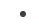首先，你将面临棘手的梯度消失问题（或相关的梯度爆炸问题），这会影响深度神经网络，并使较低层难以训练。其次，对于如此庞大的网络，训练将非常缓慢。第三，具有数百万参数的模型将会有严重的过拟合训练集的风险。

## 梯度消失/爆炸问题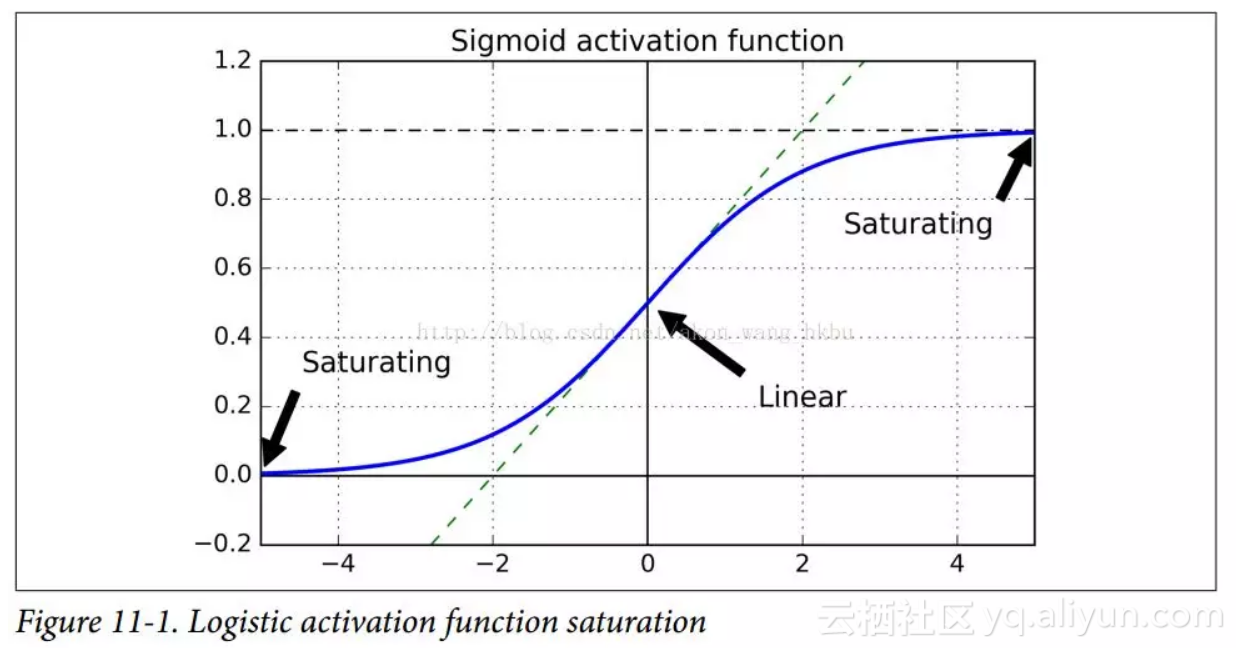Glorot 和 Bengio 在他们的论文中提出了一种显著缓解这个问题的方法。 我们需要信号在两个方向上正确地流动：在进行预测时是正向的，在反向传播梯度时是反向的。 我们不希望信号消失，也不希望它爆炸并饱和。 为了使信号正确流动，作者认为，我们需要每层输出的方差等于其输入的方差。（这里有一个比喻：如果将麦克风放大器的旋钮设置得太接近于零，人们听不到声音，但是如果将麦克风放大器设置得太大，声音就会饱和，人们就会听不懂你在说什么。 现在想象一下这样一个放大器的链条：它们都需要正确设置，以便在链条的末端响亮而清晰地发出声音。 你的声音必须以每个放大器的振幅相同的幅度出来。）而且我们也需要梯度在相反方向上流过一层之前和之后有相同的方差（如果您对数学细节感兴趣，请查阅论文）。实际上不可能保证两者都是一样的，除非这个层具有相同数量的输入和输出连接，但是他们提出了一个很好的折衷办法，在实践中证明这个折中办法非常好：随机初始化连接权重必须如公式 11-1 所描述的那样。其中n_inputsn_outputs是权重正在被初始化的层（也称为扇入和扇出）的输入和输出连接的数量。 这种初始化策略通常被称为Xavier初始化（在作者的名字之后），或者有时是 Glorot 初始化。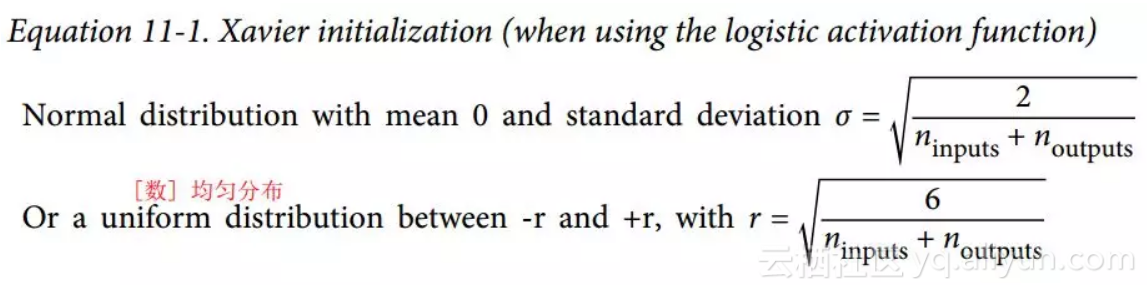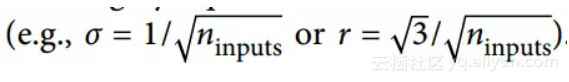我们在第 10 章中使用了这个简化的策略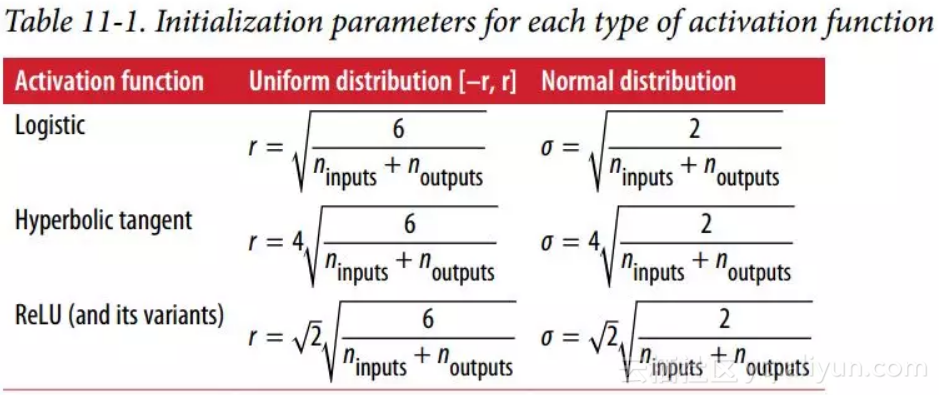he_init = tf.contrib.layers.variance_scaling_initializer()
hidden1 = tf.layers.dense(X, n_hidden1, activation=tf.nn.relu,
kernel_initializer=he_init, name="hidden1")

He 初始化只考虑了扇入，而不是像 Xavier 初始化那样扇入和扇出之间的平均值。 这也是variance_scaling_initializer()函数的默认值，但您可以通过设置参数mode ="FAN_AVG"来更改它。

## 非饱和激活函数

Glorot 和 Bengio 在 2010 年的论文中的一个见解是，消失/爆炸的梯度问题部分是由于激活函数的选择不好造成的。 在那之前，大多数人都认为，如果大自然选择在生物神经元中使用 sigmoid 激活函数，它们必定是一个很好的选择。 但事实证明，其他激活函数在深度神经网络中表现得更好，特别是 ReLU 激活函数，主要是因为它对正值不会饱和（也因为它的计算速度很快）。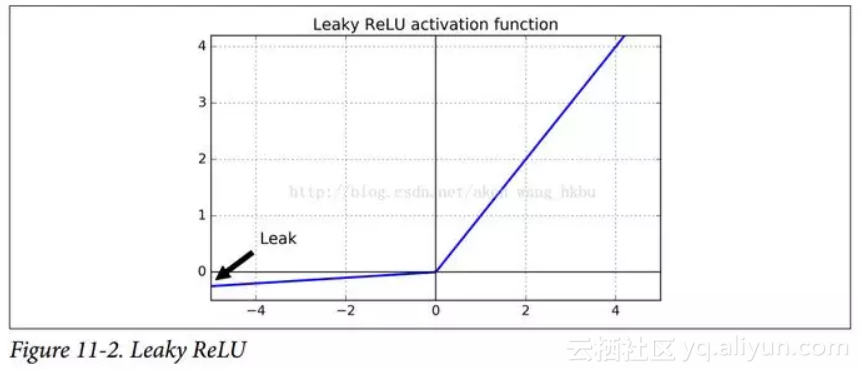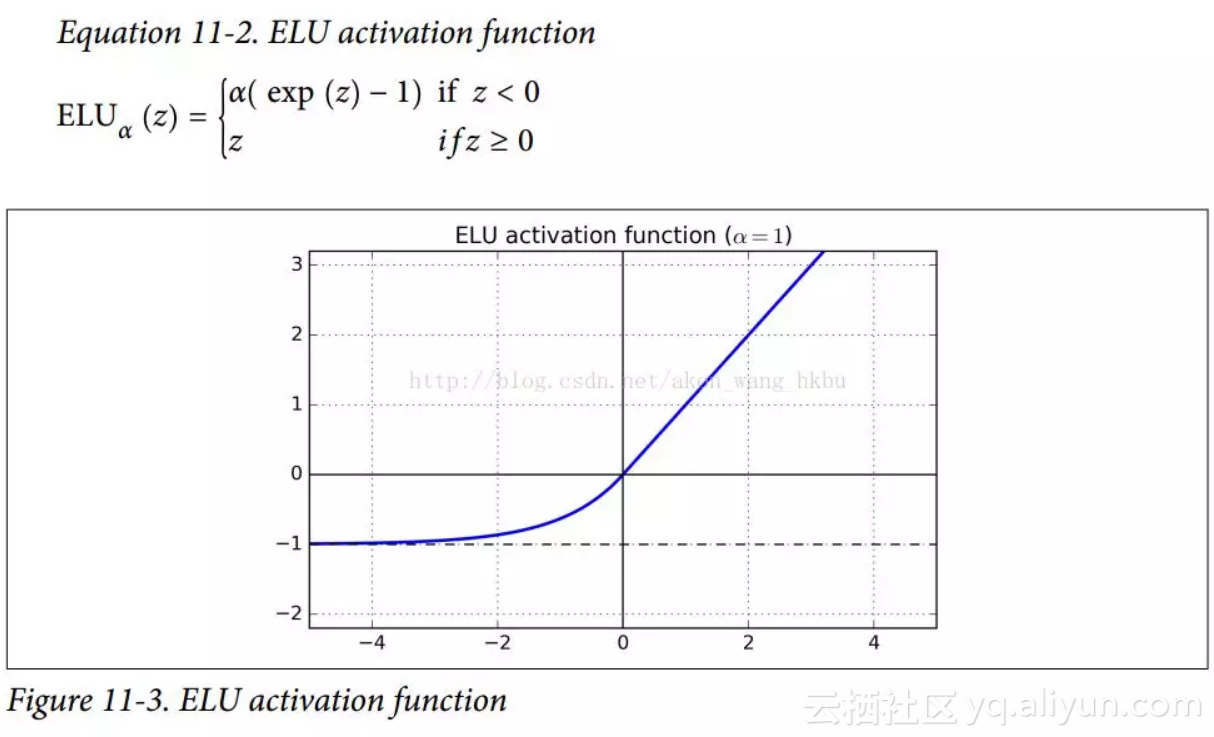首先它在z < 0时取负值，这使得该单元的平均输出接近于 0。这有助于减轻梯度消失问题，如前所述。 超参数α定义为当z是一个大的负数时，ELU 函数接近的值。它通常设置为 1，但是如果你愿意，你可以像调整其他超参数一样调整它。其次，它对z < 0有一个非零的梯度，避免了神经元死亡的问题。第三，函数在任何地方都是平滑的，包括z = 0左右，这有助于加速梯度下降，因为它不会弹回z = 0的左侧和右侧。

ELU 激活函数的主要缺点是计算速度慢于 ReLU 及其变体（由于使用指数函数），但是在训练过程中，这是通过更快的收敛速度来补偿的。 然而，在测试时间，ELU 网络将比 ReLU 网络慢。

TensorFlow 提供了一个可以用来建立神经网络的elu()函数。 调用fully_connected()函数时，只需设置activation_fn参数即可：

hidden1 = tf.layers.dense(X, n_hidden1, activation=tf.nn.elu, name="hidden1")

TensorFlow 没有针对 leaky ReLU 的预定义函数，但是很容易定义：

def leaky_relu(z, name=None):
return tf.maximum(0.01 * z, z, name=name)

hidden1 = tf.layers.dense(X, n_hidden1, activation=leaky_relu, name="hidden1")

## 批量标准化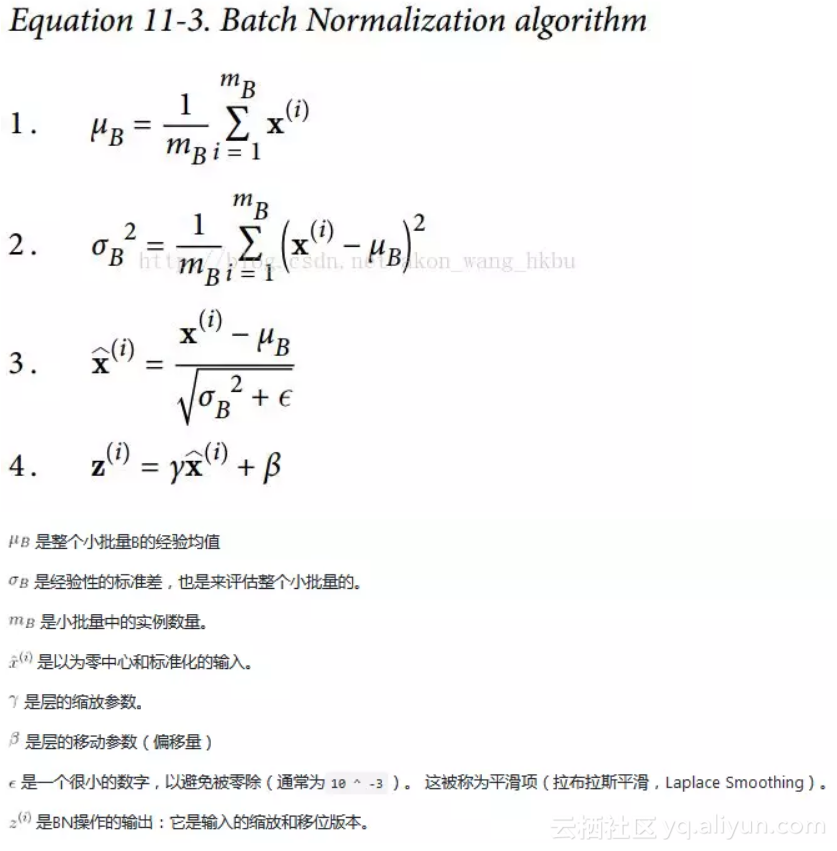TensorFlow 提供了一个batch_normalization()函数，它简单地对输入进行居中和标准化，但是您必须自己计算平均值和标准差（基于训练期间的小批量数据或测试过程中的完整数据集） 作为这个函数的参数，并且还必须处理缩放和偏移量参数的创建（并将它们传递给此函数）。 这是可行的，但不是最方便的方法。 相反，你应该使用batch_norm()函数，它为你处理所有这些。 您可以直接调用它，或者告诉fully_connected()函数使用它，如下面的代码所示：decay更名为momentumis_training被重命名为trainingupdates_collections被删除：批量标准化所需的更新操作被添加到UPDATE_OPS集合中，并且您需要在训练期间明确地运行这些操作（请参阅下面的执行阶段）我们不需要指定scale = True，因为这是默认值。

import tensorflow as tf

n_inputs = 28 * 28
n_hidden1 = 300
n_hidden2 = 100
n_outputs = 10

X = tf.placeholder(tf.float32, shape=(None, n_inputs), name="X")

training = tf.placeholder_with_default(False, shape=(), name='training')

hidden1 = tf.layers.dense(X, n_hidden1, name="hidden1")
bn1 = tf.layers.batch_normalization(hidden1, training=training, momentum=0.9)
bn1_act = tf.nn.elu(bn1)

hidden2 = tf.layers.dense(bn1_act, n_hidden2, name="hidden2")
bn2 = tf.layers.batch_normalization(hidden2, training=training, momentum=0.9)
bn2_act = tf.nn.elu(bn2)

logits_before_bn = tf.layers.dense(bn2_act, n_outputs, name="outputs")
logits = tf.layers.batch_normalization(logits_before_bn, training=training,
momentum=0.9)

X = tf.placeholder(tf.float32, shape=(None, n_inputs), name="X")
training = tf.placeholder_with_default(False, shape=(), name='training')

from functools import partial

my_batch_norm_layer = partial(tf.layers.batch_normalization,
training=training, momentum=0.9)

hidden1 = tf.layers.dense(X, n_hidden1, name="hidden1")
bn1 = my_batch_norm_layer(hidden1)
bn1_act = tf.nn.elu(bn1)
hidden2 = tf.layers.dense(bn1_act, n_hidden2, name="hidden2")
bn2 = my_batch_norm_layer(hidden2)
bn2_act = tf.nn.elu(bn2)
logits_before_bn = tf.layers.dense(bn2_act, n_outputs, name="outputs")
logits = my_batch_norm_layer(logits_before_bn)

from functools import partial
from tensorflow.examples.tutorials.mnist import input_data
import tensorflow as tf

if __name__ == '__main__':
n_inputs = 28 * 28
n_hidden1 = 300
n_hidden2 = 100
n_outputs = 10

batch_norm_momentum = 0.9
learning_rate = 0.01

X = tf.placeholder(tf.float32, shape=(None, n_inputs), name = 'X')
y = tf.placeholder(tf.int64, shape=None, name = 'y')
training = tf.placeholder_with_default(False, shape=(), name = 'training')#给Batch norm加一个placeholder

with tf.name_scope("dnn"):
he_init = tf.contrib.layers.variance_scaling_initializer()
#对权重的初始化

my_batch_norm_layer = partial(
tf.layers.batch_normalization,
training = training,
momentum = batch_norm_momentum
)

my_dense_layer = partial(
tf.layers.dense,
kernel_initializer = he_init
)

hidden1 = my_dense_layer(X ,n_hidden1 ,name = 'hidden1')
bn1 = tf.nn.elu(my_batch_norm_layer(hidden1))
hidden2 = my_dense_layer(bn1, n_hidden2, name = 'hidden2')
bn2 = tf.nn.elu(my_batch_norm_layer(hidden2))
logists_before_bn = my_dense_layer(bn2, n_outputs, name = 'outputs')
logists = my_batch_norm_layer(logists_before_bn)

with tf.name_scope('loss'):
xentropy = tf.nn.sparse_softmax_cross_entropy_with_logits(labels = y, logits= logists)
loss = tf.reduce_mean(xentropy, name = 'loss')

with tf.name_scope('train'):
training_op = optimizer.minimize(loss)

with tf.name_scope("eval"):
correct = tf.nn.in_top_k(logists, y, 1)
accuracy = tf.reduce_mean(tf.cast(correct, tf.float32))

init = tf.global_variables_initializer()
saver = tf.train.Saver()

n_epoches = 20
batch_size = 200
# 注意：由于我们使用的是 tf.layers.batch_normalization() 而不是 tf.contrib.layers.batch_norm()（如本书所述），
# 所以我们需要明确运行批量规范化所需的额外更新操作（sess.run([ training_op，extra_update_ops], ...)。
extra_update_ops = tf.get_collection(tf.GraphKeys.UPDATE_OPS)

with tf.Session() as sess:
init.run()
for epoch in range(n_epoches):
for iteraton in range(mnist.train.num_examples//batch_size):
X_batch, y_batch = mnist.train.next_batch(batch_size)
sess.run([training_op,extra_update_ops],
feed_dict={training:True, X:X_batch, y:y_batch})
accuracy_val = accuracy.eval(feed_dict= {X:mnist.test.images,
y:mnist.test.labels})
print(epoch, 'Test accuracy:', accuracy_val)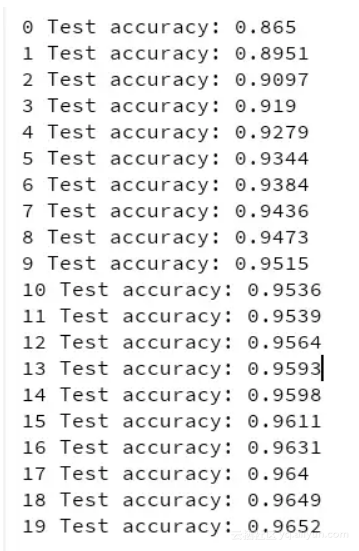with tf.name_scope("train"):
extra_update_ops = tf.get_collection(tf.GraphKeys.UPDATE_OPS)
with tf.control_dependencies(extra_update_ops):
training_op = optimizer.minimize(loss)

sess.run(training_op, feed_dict={training: True, X: X_batch, y: y_batch})

Python技术进阶交流群AI大事件 | 机器学习增强人类记忆力，谷歌上线AI速成课，TensorFlow 1.6发布
4529 0Machine Learning From Scratch-从头开始机器学习
Python implementations of some of the fundamental Machine Learning models and algorithms from scratch.
908 0TensorFlow 卷积神经网络手写数字识别数据集介绍

1551 0985 0774 0889 0TensorFlow 中文资源精选，官方网站，安装教程，入门教程，实战项目，学习路径。
Awesome-TensorFlow-Chinese TensorFlow 中文资源全集，学习路径推荐： 官方网站，初步了解。
1662 0TensorFlow 实战卷积神经网络之 LeNet

2970 01033 01339 0
47

723

+ 订阅

OceanBase 入门到实战教程